SSC  >  Logical Reasoning - Test 9

# Logical Reasoning - Test 9

Test Description

## 25 Questions MCQ Test SSC CGL Tier 1 Mock Test Series | Logical Reasoning - Test 9

Logical Reasoning - Test 9 for SSC 2022 is part of SSC CGL Tier 1 Mock Test Series preparation. The Logical Reasoning - Test 9 questions and answers have been prepared according to the SSC exam syllabus.The Logical Reasoning - Test 9 MCQs are made for SSC 2022 Exam. Find important definitions, questions, notes, meanings, examples, exercises, MCQs and online tests for Logical Reasoning - Test 9 below.
Solutions of Logical Reasoning - Test 9 questions in English are available as part of our SSC CGL Tier 1 Mock Test Series for SSC & Logical Reasoning - Test 9 solutions in Hindi for SSC CGL Tier 1 Mock Test Series course. Download more important topics, notes, lectures and mock test series for SSC Exam by signing up for free. Attempt Logical Reasoning - Test 9 | 25 questions in 15 minutes | Mock test for SSC preparation | Free important questions MCQ to study SSC CGL Tier 1 Mock Test Series for SSC Exam | Download free PDF with solutions
 1 Crore+ students have signed up on EduRev. Have you?
Logical Reasoning - Test 9 - Question 1

### Directions: In the following question, find the odd word from the given alternatives.

Detailed Solution for Logical Reasoning - Test 9 - Question 1

Turmeric, Pepper, Cuminseed are flowering plant where almond are drupe consisting of an outer hull and a hard shell with the seed, which is not a true nut, inside.
Hence, Almond is odd one among given alternatives.

Logical Reasoning - Test 9 - Question 2

### In the following question, find the odd image from the given alternatives.

Detailed Solution for Logical Reasoning - Test 9 - Question 2

Image of option 3) is different from other three alternatives as in all three images line inside square pointing toward corner where total three line intersect but in option 3) line inside square point in corner where only two line intersect.
Hence, option 3) is correct option.

Logical Reasoning - Test 9 - Question 3

### Find the missing term in diagram shown below?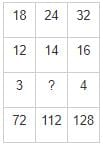Detailed Solution for Logical Reasoning - Test 9 - Question 3

By observing above given matrix,
⇒ 18 × (12/3) = 12 × 6 = 72
⇒ 32 × (16/4) = 32 × 4 = 128
⇒ 24 × (14/?) = 112
⇒ (24 × 14) ÷ 112 = ?
⇒ ? = 3
Hence, the missing number is “3”

Logical Reasoning - Test 9 - Question 4

Directions: In the following question, find the odd one from the given alternatives.

Detailed Solution for Logical Reasoning - Test 9 - Question 4

1) 7 : 19 ⇒ (7 × 3) - 2 = 19
2) 5 : 13 ⇒ (5 × 3) - 2 = 13
3) 11 : 31 ⇒ (11 × 3) - 2 = 31
4) 15 : 43 ⇒ (15 × 3) - 2 = 43
If we multiply first number by 3 and then 2 subtracted from number then number will became prime number.
Therefore, 5 : 13 is odd one among given alternatives.

Logical Reasoning - Test 9 - Question 5

Directions: In the following questions, select the related word from the given alternatives.
Evaporation : Cloud : Rain : : ?

Detailed Solution for Logical Reasoning - Test 9 - Question 5

Evaporation of water occurs when the surface of the liquid is exposed, allowing molecules to escape and form water vapor; this vapor can then rise up and form clouds. Similarly, When an Accident happen then there is an Injury and causes Pain.
Hence, “Accident : Injury : Pain” is correct option.

Logical Reasoning - Test 9 - Question 6

Directions: In the following question, you have to identify the correct response from the given premises stated according to following symbols.
If > is +, V is x, < is -, ∧ is ÷ , + is =, x is <, and - is >

Detailed Solution for Logical Reasoning - Test 9 - Question 6

Based on condition given,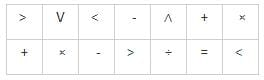1) 6 > 2 > 3 < 8 ∨ 4 + 13
⇒ 6 + 2 + 3 - 8 × 4 = 13
⇒ - 21 = 13 (false)
2) 6 ∧ 2 < 3 > 8 < 4 - 13
⇒ 6 ÷ 2 - 3 + 8 - 4 > 13
⇒ 4 > 13 (false)
3) 6 ∨ 2 < 4 ∧ 8 > 4 × 13
⇒ 6 × 2 - 4 ÷ 8 + 4 < 13
⇒ 15.5 < 13 (false)
4) 5 > 2 ∨ 3 > 8 ∧ 4 + 13
⇒ 5 + 2 × 3 + 8 ÷ 4 = 13
⇒ 13 = 13 (True)

Logical Reasoning - Test 9 - Question 7

In the following questions, select the related number from the given alternatives.
419 : 213 : : 911 : ?

Detailed Solution for Logical Reasoning - Test 9 - Question 7

In 419 : 213
Square root of 4 = 2
Square root of 1 = 1
Square root of 9 = 3
Hence, 213 is square root of each digit of 419. Similarly, 311 is square root of each digit of 911.
Hence, ‘311” is correct option.

Logical Reasoning - Test 9 - Question 8

Directions: In the following question, find the odd letters from the given alternatives.

Detailed Solution for Logical Reasoning - Test 9 - Question 8

1) UTSR ⇒ it is an reverse order of consecutive letters RSTU of English alphabet.
2) IHGE ⇒ it is an reverse order of letters EGHI (not consecutive) of English alphabet.
3) NMLK ⇒ it is an reverse order of consecutive letters KLMN of English alphabet.
4) ZYXW ⇒ it is an reverse order of consecutive letters WXYZ of English alphabet.
Hence, “IHGE” is odd one among given alternatives.

Logical Reasoning - Test 9 - Question 9

In a code language, DISTANCE is written as IDSUBECN and DOCUMENT is written as ODCVNTNE. How is THURSDAY written in that language?

Detailed Solution for Logical Reasoning - Test 9 - Question 9

DISTANCE is written as IDSUBECN and DOCUMENT is written as ODCVNTNE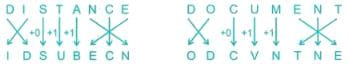Similarly,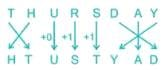Hence, THURSDAY written in that language as “HTUSTYAD”

Logical Reasoning - Test 9 - Question 10

A watch reads 3:30, If the minute hand towards the north – east direction, then in what direction will the hour hand point.

Detailed Solution for Logical Reasoning - Test 9 - Question 10

Clearly to show 3.30, the position of the minute and hour hands of the clock will be as shown,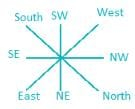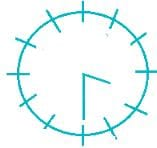As we can see at 3:30 hour hand will be at less 90° but more than with respect to minute other hence if minute hand is facing north – east then hour hand will be at same direction North – West.

Logical Reasoning - Test 9 - Question 11

Arrange the given words in the sequence in which they occur in the dictionary.
i. Irrelevant
ii. Invincible
iii. Irresistible
iv. Invariable
v. Investigate

Detailed Solution for Logical Reasoning - Test 9 - Question 11

Arranging given word based on dictionary,
iv. Invariable
v. Investigate
ii. Invincible
i. Irrelevant
iii. Irresistible
order in which now they will appear as per dictionary will be iv, v, ii, i, iii

Logical Reasoning - Test 9 - Question 12

Rearrange the jumbled letters to make meaningful words and then select the one which is different.

Detailed Solution for Logical Reasoning - Test 9 - Question 12

DOGL → GOLD
TSEVO → STOVE
ENZROB → BRONZE
LVREIS → SILVER
GOLD, BRONZE, SILVER are metals but STOVE isn’t a metal.
So, STOVE is different among the given words.

Logical Reasoning - Test 9 - Question 13

Introducing a man, a woman said, His wife is the only daughter of my mother”. How is the woman related to the man?

Detailed Solution for Logical Reasoning - Test 9 - Question 13

Table is drawn describing symbols and their meanings: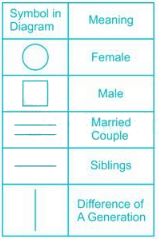His wife is the only daughter of my mother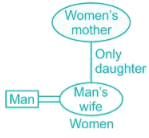Hence, Women is wife of Man she is taking about here.

Logical Reasoning - Test 9 - Question 14

The following diagram represent people who speak different languages.
i) Konkani
ii) Hindi
iv) English
What does the shaded area represents?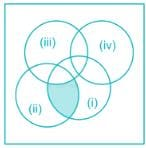Detailed Solution for Logical Reasoning - Test 9 - Question 14

The shaded region highlights the area of intersection between i) and ii).Since i) is Konkani and ii) is Hindi, the area of intersection will be those who speak both these languages.

Logical Reasoning - Test 9 - Question 15

Directions: In the following question, two statements are given each followed by two conclusions I and II. You have to consider the statements to be true even if they seem to be at variance from commonly known facts. You have to decide which of the given conclusions, if any, follows from the given statements.
Statements:
(I) The DRDO has tested the Smart Anti Airfield Weapon from an Indian Air Force aircraft.
(II) The lightweight high precision guided bomb is one of the world class weapons systems.
Conclusions:
(I) DRDO Chairman Dr. S. Christopher congratulated the DRDO and the IAF teams for the successful mission.
(II) The test was carried out by IAF's Aircraft and System Testing Establishment.

Detailed Solution for Logical Reasoning - Test 9 - Question 15

→ Option 3) is correct, means neither I nor II conclusion follow.
→ The conclusions provided do not contain any information which supports the success of the mission. Conclusion I talks about the DRDO Chairman congratulating the DRDO & IAF teams, however, the sentence does not provide any information stating whether the mission was a success or a failure.
Conclusion II talks about the teams involved in the mission, however, absolute absence of any of such information negates this conclusion too.
Hence, we say that neither I nor II follow the given statements.

Logical Reasoning - Test 9 - Question 16

Directions: In question below two statements are given, followed by two conclusions I and II. You have to consider the statements to be true even if they seem to be at variance from commonly known facts. You have to decide which of the given conclusions, if any, follow form the given statements.
Statements:
1) All doctors have good health.
2) Some employees are doctors.
Conclusions:
I. All employees have good health follow.
II. Some men with good health are not doctors.

Detailed Solution for Logical Reasoning - Test 9 - Question 16

Drawing least possible Venn diagram,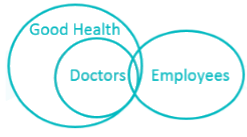Conclusions:
I. All employees have good health: Does not follow.
II. Some men with good health are not doctors: This case is not definite as all good health people can be doctor. Thus this conclusion is false.
Hence none of the conclusions follows.

Logical Reasoning - Test 9 - Question 17

Out of the six members’ panel sitting in a row, U is to the left of V but to the right of W, who is to the right of X, and Y is at the right of Z, who’s to the left of X. Find the members sitting right in the middle?

Detailed Solution for Logical Reasoning - Test 9 - Question 17

Members: 6
1. U is to the left of V but to the right of W, who is to the right of X ⇒ this gives 3 possibilities: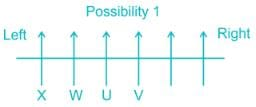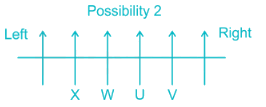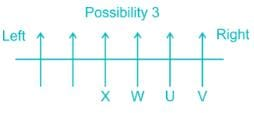2. Y is at the right of Z, who’s to the left of X. ⇒ This eliminates 1st and 2nd possibility: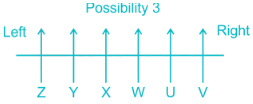Clearly, X and W are sitting in the middle.

Logical Reasoning - Test 9 - Question 18

A series is given with one term missing. Choose the correct alternative from the given ones that will complete the series.
Glacier, Waterfall, River, ?

Detailed Solution for Logical Reasoning - Test 9 - Question 18

Above given series depicts the lifecycle of fresh water.
The glacier melts and forms waterfall. Waterfall becomes steady and forms river which ultimately flows into the sea.

Logical Reasoning - Test 9 - Question 19

In the following question, which one set of letters when sequentially placed at the gaps in the given letter series shall complete it?
a_c_a_cb_ccb

Detailed Solution for Logical Reasoning - Test 9 - Question 19

1) cbca ⇒ accb/accb/accb (repeated pattern of “accb”)
2) abcd ⇒ aacbaccbdccb
3) bdac ⇒ abcdaacbcccb
4) abdc ⇒ aacbadcbcccb
Therefore, “cbca” are set of letters which will complete the series.

Logical Reasoning - Test 9 - Question 20

A series is given with one term missing. Select the correct alternative from the given ones that will complete the series.
1000, 1100, 990, ?, 980.1

Detailed Solution for Logical Reasoning - Test 9 - Question 20

The pattern here is,
1000 × 1/10 = 100 ⇒ 1000 + 100 = 1100
1100 × 1/10 = 110 ⇒ 1100 – 110 = 990
990 × 1/10 = 99 ⇒ 990 + 99 = 1089
1089 × 1/10 = 108.9 ⇒ 1089 – 108.9 = 980.1
Hence the missing number is 1089.

Logical Reasoning - Test 9 - Question 21

PON, RQP, TSR,VUT, ___, ___

Detailed Solution for Logical Reasoning - Test 9 - Question 21

It is reverse order of alphabetical letters in the following way,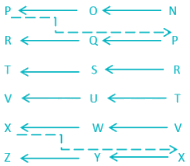Hence the next terms are XWV and ZYX.

Logical Reasoning - Test 9 - Question 22

A cube, painted with a certain colour (Red) on the outer surface, is of 2 inches height, 2 inches wide and 2 inches across. If it’s cut into one-inch cubes as shown by dotted lines, indicate the number of cubes having colour (Red) at least on two sides?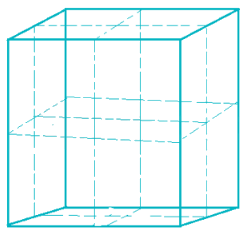Detailed Solution for Logical Reasoning - Test 9 - Question 22

Volume of cuboid = L × B × H = 2 × 2 × 2 = 8
Volume of Cuboid = Number of small cubes × Volume of 1 cube of 1 inch dimensions
8 = Number of cubes × 1 [as dimensions are 1 each so volume will be 1×1×1 = 1 cubic inch]
⇒ Number of cubes = 8
The given cuboid will be cut into 8 cubes. We’ve to find how many cubes out of these eights will have red on 2-sides.
Well, these eight cubes will be identical to each other because each of these 8 cubes will have ‘one side face’, ‘one front/back face’ and ‘one top/bottom face’ and ‘three inner faces’.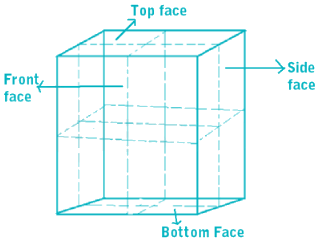And, because Cuboid is coated red on outer surface so each of the Front/back face, side face and top/bottom face would also be coated red.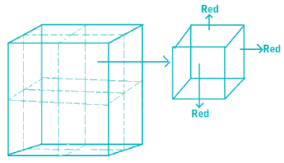⇒ Each cube would have 3 faces as red.
⇒ 8 cubes have red on two-sides.

Logical Reasoning - Test 9 - Question 23

Direction: In the following question, which answer figure will complete the pattern in the question figure?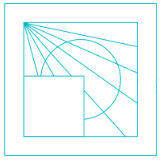Detailed Solution for Logical Reasoning - Test 9 - Question 23

Two lines should pass through the circle and one line from outside the circle from left top corner towards right bottom corner. As per these two statements, option 3 is the correct answer.

Logical Reasoning - Test 9 - Question 24

Identify the answer figure from which the pieces given in the question figure have been cut.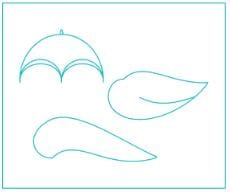Detailed Solution for Logical Reasoning - Test 9 - Question 24

Clearly option 1 is the answer.

Logical Reasoning - Test 9 - Question 25

From the given answer figures, select the figure which is hidden / embedded in the question figure.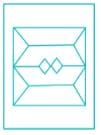Detailed Solution for Logical Reasoning - Test 9 - Question 25

From the given figure it is very clear that only option D figure can be found in the center of the given question figure.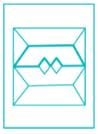## SSC CGL Tier 1 Mock Test Series

42 docs|103 tests
 Use Code STAYHOME200 and get INR 200 additional OFF Use Coupon Code
Information about Logical Reasoning - Test 9 Page
In this test you can find the Exam questions for Logical Reasoning - Test 9 solved & explained in the simplest way possible. Besides giving Questions and answers for Logical Reasoning - Test 9, EduRev gives you an ample number of Online tests for practice

## SSC CGL Tier 1 Mock Test Series

42 docs|103 tests